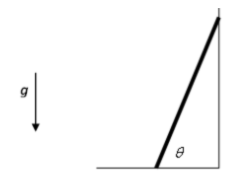# Problem: A ladder, length L, with mass M, leans against a frictionless wall at an angle of θ = 70° as shown.If the ladder is in static equilibrium, A. The net force is zero but the net torque is not. B. The net torque is zero but the net force is not. C. The net force and net torque are both zero.D. Neither net force nor net torque is zero.

###### FREE Expert Solution

A rigid body is said to be in equilibrium when both its linear and angular acceleration are zero. Static equilibrium implies an object is at rest while dynamic equilibrium implies that an object is moving at constant velocity.

97% (374 ratings)###### Problem Details

A ladder, length L, with mass M, leans against a frictionless wall at an angle of θ = 70° as shown.If the ladder is in static equilibrium,

A. The net force is zero but the net torque is not.

B. The net torque is zero but the net force is not.

C. The net force and net torque are both zero.

D. Neither net force nor net torque is zero.